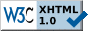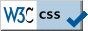Quick Navigation Bar introduction :: data structures :: program control and functions [ toc | forums ] Note: If the document URL does not begin with https://randu.org/tutorials/perl/ then you are viewing a copy. Please direct your browser to the correct location for the most recent version.

# Data Structures: Scalars, Arrays, Hashes

• In this section, we'll discuss the basic data structures available in Perl. Make sure you practice some of the examples discussed here!

## Data Structures :: Scalars

• A scalar is a number or a string. You can apply the usual manipulations on numbers, +, -, *, /, %, but Perl also has an exponentional operator. So how would you write 2 cubed?
```  2**3
```
Powerful? Yes.
• What about string operations? String concatenation for example:
```  "hello" . "world"
```
The above would result in a single word, "helloworld". You can string multiple concatenations into one operation.
• There is a string repetition operator: `x`. For example:
```  "w00t" x 3
```
would yield "w00tw00tw00t".
• The standard logical operators are present and can be used on strings too! The following table (Schwartz 37) illustrates the Logical operators on scalars:  Comparison Numeric String Equal == eq Not Equal != ne Less Than < lt Greater Than > gt Less Than or Equal To <= le Greater Than or Equal To >= ge
• Other operators include ```++, --, !, &&, ||, ?:, *= += -=, ->, \```. See Schwartz pgs. 38-39.
• One of the powerhouses of Perl is the automatic type conversion between numbers and strings. It technically isn't even a type conversion because numbers and strings are both scalars.
```  (4**2 + 3 / 2) . " is the result of the expression 4^2 + 3/2"
```
This would result in a string "17.5 is the result of the expression 4^2 + 3/2".
• So how do we store these scalars into actual variables we can use? Simple:
```  \$result = (4**2 + 3 / 2) . " is the result of the expression 4^2 + 3/2";
```
Now the string is stored in the variable `result`.
• You can do many cool things with variables in Perl, for example:
```  (\$x, \$y) = (\$y, \$x);
```
swaps variables x and y. A far cry from C...
• To remove trailing newlines from strings, you can use either `chop` or `chomp`. `chop` always removes the last character from the string, while `chomp` only removes newlines. So be safe and use `chomp` to remove newlines from input (more on input later).

## Data Structures :: Arrays

• An array variable is signified by the `@` character.
• A list literal is defined by parentheses and separated by commas:
```  @arr_1 = (1, 2, 3);
@str_arr = ("hello", "cruel", "world");
@arr_42 = qw(the meaning of life doesn\'t exist);
@arr_gen = (0 .. 5);
```
`str_arr` is an array of strings. What is `arr_42`? It is the shorthand method of writing a list of strings using the `qw` or quote word function. `arr_gen` is the same as the list (0, 1, 2, 3, 4, 5).
• What about adding into an array or deleting from an array?
```  @arr_gen = (-1, @arr_gen, 6);   # adds -1 to the front, 6 to the back of list
(\$a, @arr_gen) = @arr_gen;      # removes -1 from @arr_gen to \$a
\$length = @arr_gen;             # length is 7
(\$first) = @arr_gen;
```
These aren't the only ways to add or remove from a list (see below). What does the fourth statement do? Whenever you set an array to a scalar, Perl automatically takes the length of the array and sets that value to the scalar. The last statement gets the first element of `arr_gen` or 0. But how do we access different elements in the array?
• Element access in arrays are much like any other programming language:
```  \$arr_gen = 42;
@arr_gen[0,1] = (10, 11);
```
In the first line, we access the fourth element in the array and change the value to 42. In the second line, we change the first two elements in the array to 10 and 11 by slicing. You can easily reverse lists using slicing also (see Schwartz pg. 53), but there is an even easier method below for list reversals.
• Another powerful feature of arrays in Perl is negative indexing:
```  @arr_gen = (0 .. 5);
\$a = \$arr_gen[-2];    # \$a gets assigned 4
\$last = \$#arr_gen;    # last == 4
```
What does the last statement do? It gives `last` the last index value of the array (not the length).
• So how do we add/remove from lists with ease? Simple, treat them like stacks or queues:
```  @arr = (1 .. 3);
\$new_rvalue = 4;
\$new_lvalue = 0;
push(@arr, \$new_rvalue);       # @arr == (1, 2, 3, 4)
unshift(@arr, \$new_lvalue);    # @arr == (0, 1, 2, 3, 4)
\$x = pop(@arr);                # \$x == 4, @arr == (0, 1, 2, 3)
\$y = shift(@arr);              # \$y == 0, @arr == (1, 2, 3)
```
Instant stacks and queues. Nice!
• How about reversing a list? Simply call the reverse function:
```  @rev_a = reverse(@a);
```
Simple enough... how about sorting a list?
```  @mylist = (1, 2, 4, 0, 32, 22, 17, 53, 42);
@sorted = sort(@mylist);
```
`@sorted` is 0, 1, 17, 2, 22, 32, 4, 42, 53. Wait! That isn't sorted... well technically it is. The `sort` function performs an ASCII sort, not a numeric sort. More on sorting numerically later.

## Data Structures :: Hashes

• A hash is a data structure that holds scalars, but is indexed by a key value or another scalar.
• Hash variables are declared with `%`. Elements in the hash are referenced by `\$key`. For example:
```  \$myhash{"key1"} = "555-111-29391-secret-key!"
\$myhash{"key2"} = "333-123-33904-secret-key!"
\$key = "key2";
print "\$myhash{\$key}";         # Prints the value in the hash of key2
```
• There are some useful hash functions:
• keys - lists all current keys in the hash (order doesn't matter. Why? So a useful way to use the `keys` function call is to loop through each key in the hash perhaps:
```  foreach \$key (keys (%myhash)) {
print "\$myhash{\$key}\n";
}
```
This would loop through the `%myhash` hash and print each key. More on `foreach` later...
• values - returns a list of all values in the hash.
• each - `each` works much like keys does, but returns a list of both the key and the hash value.
• delete - deletes a value out of the hash:
```  delete \$myhash{"key1"};         # Deletes the key key1 and it's value
```
• Hashes can be sliced also! For example:
```  @keys = qw(key3 key4 key5);
@myhash{@keys} = ("665-345-09284-secret-key!", "958-544-94950-secret-key!",
"448-39403-secret-key!");
```
`@myhash{@keys}` is the same as ```@myhash{"key3", "key4", "key5"} = ("665-345-09284-secret-key!", "958-544-94950-secret-key!", "448-39403-secret-key!");```
• ``` ```
``` Data Structures Review Scalars are just numbers or strings. It's a fundamentally supported data type in Perl! Arrays are collections of scalars indexed by integers much like other programming languages, but Perl has powerful extras added in. Hashes are like arrays, but with scalar indexes. There are other data structures, custom records and self-referential types. Notice: Please do not replicate or copy these pages and host them elsewhere. This is to ensure that the latest version can always be found here. Disclaimer: The document author has published these pages with the hope that it may be useful to others. However, the document author does not guarantee that all information contained on these webpages are correct or accurate. There is no warranty, expressed or implied, of merchantability or fitness for any purpose. The author does not assume any liability or responsibility for the use of the information contained on these webpages. If you see an error, please send an email to the address below indicating the error. Your feedback is greatly appreciated and will help to continually improve these pages. © 1999-2023 Alfred Park (fred [ANTISPAM-REMOVE-THIS] AT randu.org)```